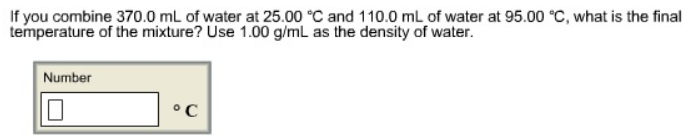Chemistry Calorimetry Solution: If you combine 370.0 mL of water at 25.00 ° C and ...

# Solution: If you combine 370.0 mL of water at 25.00 ° C and 110.0 mL of water at 95.00 °C. what is the final temperature of the mixture? Use 1.00 g/mL as the density of water.

Problem

If you combine 370.0 mL of water at 25.00 ° C and 110.0 mL of water at 95.00 °C. what is the final temperature of the mixture? Use 1.00 g/mL as the density of water.View Complete Written Solution